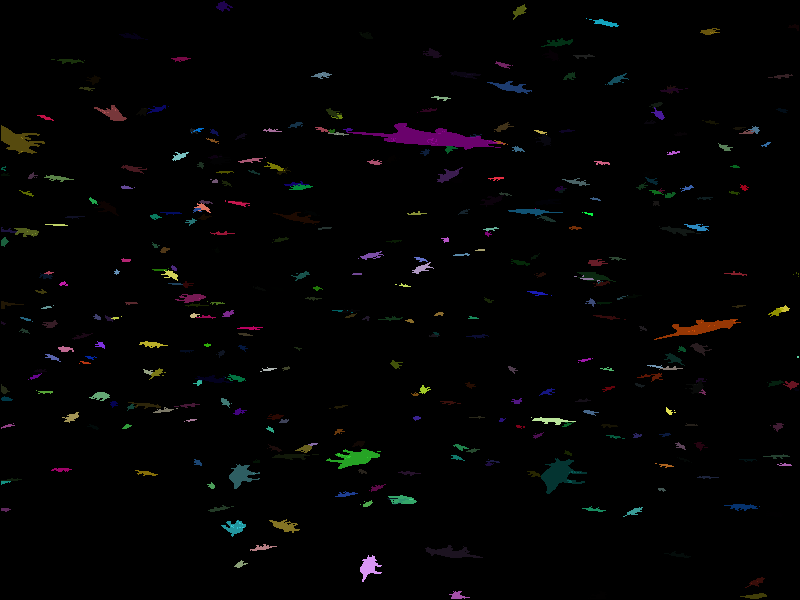# Instanced rendering of arbitrarily transformed meshes#```from vispy import app, gloo, visuals, scene, use
import numpy as np
from scipy.spatial.transform import Rotation

# full gl+ context is required for instanced rendering
use(gl='gl+')

// these attributes will be defined on an instance basis
attribute vec3 shift;
attribute vec4 color;
attribute vec3 transform_x;
attribute vec3 transform_y;
attribute vec3 transform_z;

varying vec4 v_color;

void main() {
v_color = color;
// transform is generated from column vectors (new basis vectors)
// https://en.wikibooks.org/wiki/GLSL_Programming/Vector_and_Matrix_Operations#Constructors
mat3 instance_transform = mat3(transform_x, transform_y, transform_z);
vec3 pos_rotated = instance_transform * \$position;
vec4 pos_shifted = vec4(pos_rotated + shift, 1);
gl_Position = \$transform(pos_shifted);
}
"""

varying vec4 v_color;

void main() {
gl_FragColor = v_color;
}
"""

class InstancedMeshVisual(visuals.Visual):
def __init__(self, vertices, faces, positions, colors, transforms, subdivisions=5):

self.set_gl_state('translucent', depth_test=True, cull_face=True)
self._draw_mode = 'triangles'

# set up vertex and index buffer
self.vbo = gloo.VertexBuffer(vertices.astype(np.float32))
self.shared_program.vert['position'] = self.vbo
self._index_buffer = gloo.IndexBuffer(data=faces.astype(np.uint32))

# create a vertex buffer with a divisor argument of 1. This means that the
# attribute value is set to the next element of the array every 1 instance.
# The length of the array multiplied by the divisor determines the number
# of instances
self.shifts = gloo.VertexBuffer(positions.astype(np.float32), divisor=1)
self.shared_program['shift'] = self.shifts

# vispy does not handle matrix attributes (likely requires some big changes in GLIR)
# so we decompose it into three vec3; (column vectors of the matrix)
transforms = transforms.astype(np.float32)
self.transforms_x = gloo.VertexBuffer(transforms[..., 0].copy(), divisor=1)
self.transforms_y = gloo.VertexBuffer(transforms[..., 1].copy(), divisor=1)
self.transforms_z = gloo.VertexBuffer(transforms[..., 2].copy(), divisor=1)
self.shared_program['transform_x'] = self.transforms_x
self.shared_program['transform_y'] = self.transforms_y
self.shared_program['transform_z'] = self.transforms_z

# we can provide additional buffers with different divisors, as long as the
# amount of instances (length * divisor) is the same. In this case, we will change
# color every 5 instances
self.color = gloo.VertexBuffer(colors.astype(np.float32), divisor=1)
self.shared_program['color'] = self.color

def _prepare_transforms(self, view):
view.view_program.vert['transform'] = view.get_transform()

# create a visual node class to add it to the canvas
InstancedMesh = scene.visuals.create_visual_node(InstancedMeshVisual)

# set up vanvas
canvas = scene.SceneCanvas(keys='interactive', show=True)
view.camera = 'arcball'
view.camera.scale_factor = 1000

N = 1000

vertices, faces, _, _ = read_mesh(mesh_file)

np.random.seed(0)
pos = (np.random.rand(N, 3) - 0.5) * 1000
colors = np.random.rand(N, 4)
transforms = Rotation.random(N).as_matrix()

multimesh = InstancedMesh(vertices * 10, faces, pos, colors, transforms, parent=view.scene)
# global transforms are applied correctly after the individual instance transforms!
multimesh.transform = visuals.transforms.STTransform(scale=(3, 2, 1))

if __name__ == '__main__':
import sys
if sys.flags.interactive != 1:
app.run()
```

Total running time of the script: (0 minutes 3.797 seconds)

Gallery generated by Sphinx-Gallery